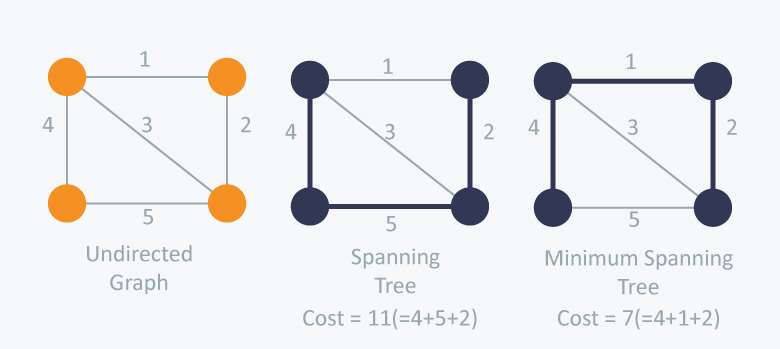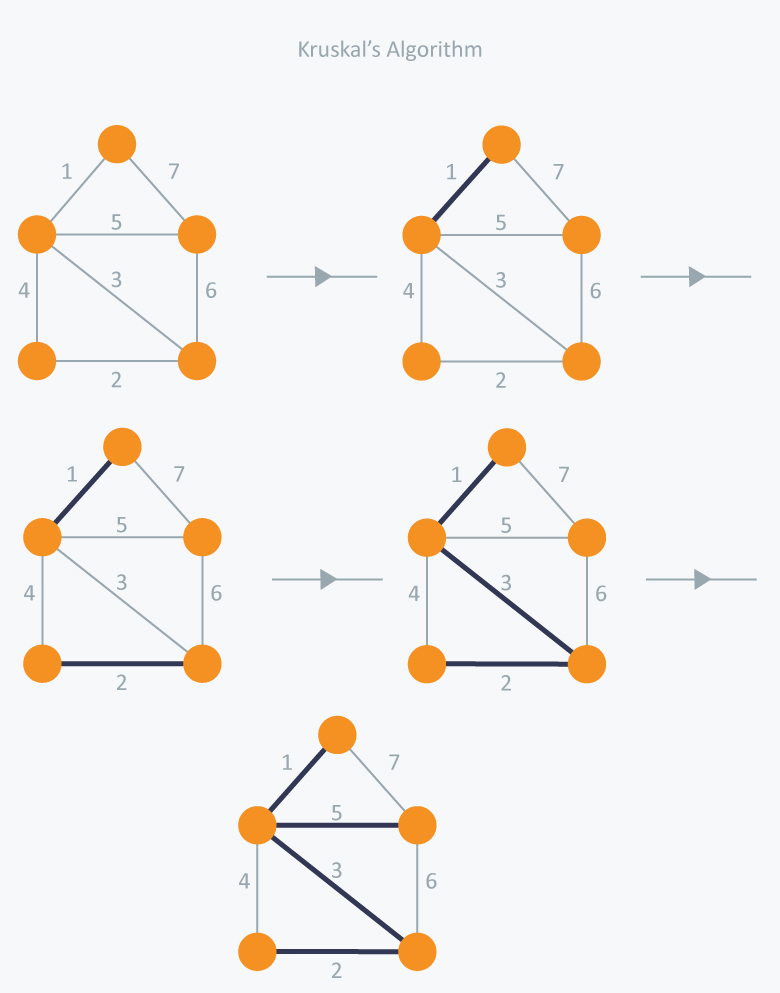# Tree Data Structure

## Spanning Trees

A spanning tree is a sub-graph of an undirected connected graph, which includes all the vertices of the graph with a minimum possible number of edges. If a vertex is missed, then it is not a spanning tree. The edges may or may not have weights assigned to them.### Minimum Spanning Tree

A minimum spanning tree is a spanning tree with the minimum possible sum of edge weights. The edges may or may not have weights assigned to them.### Finding Minimum Spanning Tree

There are many algorithms to find the minimum spanning tree. The most common ones are:

• Kruskal’s Algorithm

• Prim’s Algorithm

### Kruskal’s Algorithm

Kruskal’s algorithm is a greedy algorithm that finds a minimum spanning tree for a connected weighted graph. This means it finds a subset of the edges that forms a tree that includes every vertex, where the total weight of all the edges in the tree is minimized. If the graph is not connected, then it finds a minimum spanning forest (a minimum spanning tree for each connected component).

Algorithm Steps:

• Sort the graph edges with respect to their weights.

• Start adding edges to the MST from the edge with the smallest weight until the edge of the largest weight.

• Only add edges which doesn’t form a cycle , edges which connect only disconnected components.### Union Find Data Structure

Union Find is a data structure that keeps track of a set of elements partitioned into a number of disjoint (non-overlapping) subsets. It supports two operations:

• Find: Determine which subset a particular element is in. This can be used for determining if two elements are in the same subset.

• Union: Join two subsets into a single subset.

## Amortized Analysis

Amortized analysis is a method of analyzing the costs associated with a data structure that averages the worst operations out over time.